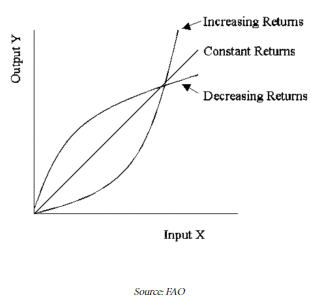# Returns to Scale and Cobb Douglas Function: With Diagrams & Examples

The compilation of these Production and Costs Notes makes students exam preparation simpler and organised.

What are returns to scale and what are its three types? Let us understand each case with a diagram for the production function. We will also learn about the famous Cobb-Douglas production function. Let us get started!

### Returns to Scale

The long-run refers to a time period where the production function is defined on the basis of variable factors only. No fixed factors exist in the long run and all factors become variable. Thus, the scale of production can be changed as inputs are changed proportionately. Thus, returns to scale are defined as the change in output as factor inputs change in the same proportion. It is a long-run concept.

### Types of Returns to Scale

There are three defined types of returns to scales, which include:

Increasing Returns to Scale
When the output increases more than proportionately when all the inputs increase proportionately, it is known as increasing returns to scale. This represents a kind of decreasing the cost to the firm. External economies of scale might be one of the reasons behind such an increase in output in increasing returns to scale. Thus, when inputs double, output more than doubles in this case.

Decreasing Returns to Scale
When the output increases less than proportionately as all the inputs increase proportionately, we call it decreasing returns to scale or diminishing returns to scale. In this case, internal or external economies are normally overpowered by internal or external diseconomies. Thus, if we double the inputs, the output will increase but by less than double.

Constant Returns to Scale
When the output increases exactly in proportion to an increase in all the inputs or factors of production, it is called constant returns to scale. For example, if twice the inputs are used in production, the output also doubles. Thus, constant returns to scale are reached when internal and external economies and diseconomies balance each other out.

A regular example of constant returns to scale is the commonly used Cobb-Douglas Production Function (CDPF). The figure given below captures how the production function looks like in the case of increasing/decreasing and constant returns to scale.### Cobb-Douglas Production Function

As we know, a production function explains the functional relationship between inputs (or factors of production) and the final physical output. Let us begin with a simple form a production function first –

Q = f(L, K)

The above mathematical equation tells us that Q (output) is a function of two inputs (assumption). These inputs are L (amount of labour) and K (hours of capital). Basing our understanding of the function above, we can now define a more specific production function – the Cobb Douglas Production Function.

Q = A Lαβ Kα

Here, Q is the output, and L and K represent units of labour and capital respectively. A is a positive constant (also called the technology coefficient). α and β are constants lying between 0 and 1.

We can calculate the Marginal Product for the CDPF and derive interesting results. Marginal Product captures the change in output due to an infinitesimal change in an input. It is calculated by the first-order differentiation of the CDPF. Hence,

MPL = Aβ Lβ-1 Kα , and MPK = Aα Lβ Kα-1

Let us now find out the implications of returns to scale on the Cobb-Douglas production function: If we are to increase all inputs by ‘c’ amount (c is a constant), we can judge the impact on output as under.

Q (cL, cK) = A (cL)β (cK)α = Acβ cα Lβ Kα = Acα+β Lβ Kα

Note that if α + β > 1 there will be increasing returns to scale.
If α + β < 1 there will be decreasing returns to scales.
And, if α + β = 1 there will be constant returns to scale (case of linear homogenous CDPF).
Thus, depending on the nature of the CDPF, there will be increasing, decreasing, or constant returns to scale.

Example:

Question:
What is the shape of the production in case of constant returns to scale?